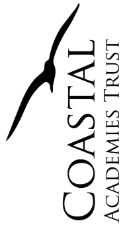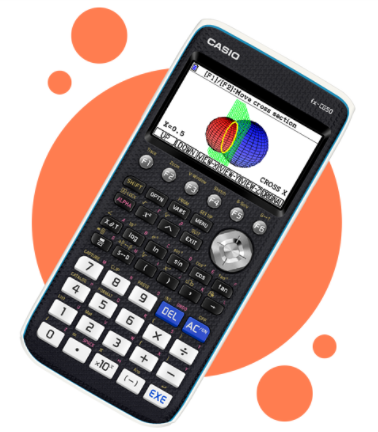﻿ IB Mathematics - King Ethelbert School# IB Mathematics

#### Course Outline

This course is designed for students who enjoy describing the real world and solving practical problems using mathematics, those who are interested in harnessing the power of technology alongside exploring mathematical models and enjoy the more practical side of mathematics.

Students we expect to follow this course are those who will not require mathematics as a major component in their future studies/career plan. It is expected that those choosing this standard level would have achieved a minimum of a grade 5 at GCSE mathematics.#### Why Study IB Maths?

The course  is aimed at students with a wide variety of mathematical backgrounds and allows students to develop a more sophisticated understanding of Mathematics with a particular focus on its application to real life problems. A significant part of the course is concerned with statistical techniques to understand and interpret data.

This standard level course consists of topics such as:

Sequences and series, Financial calculations, Proof, Modelling, Trigonometry, Voronoi diagrams, Correlation and regression, Statistical distributions and Basic Calculus.

The Graphical Display Calculator (GDC)  enables you to plot and analyse graphs and their transformations, import data directly over USB, plot data charts and calculate statistics, calculate and visualise probability distributions, plot and solve graphs in 3D, calculate matrix and vector arithmetic, perform complex calculations not possible on a scientific calculator, calculate spreadsheets and recursive sequences, and whole host more.

This calculator is required for students to access the Mathematics course#### Assessment

 Internal Assessment Exploration A piece of self chosen mathematical research 20% 20 marks External Assessment Paper 1 Short response questions (Graphical calculator required) 40% 90 minutes 80 marks Paper 2 Longer, multi-step questions (Graphical calculator required) 40% 90 minutes 80 marks

#### Entry Requirements

It is expected that those choosing this subject would have achieved a minimum of a grade 5 at GCSE mathematics

#### Contact Details for lead teacher

Mrs Gina Wilkinson